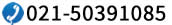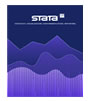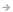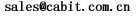联系我们销售咨询产品咨询淘宝店铺售后支持400-621-1085021-50391085sales@cabit.com.cn

 快速链接 概述最新更新：8.4下载试用文档下载

 相关软件 AMOSBILOG-MGEQSEndNoteFacetsflexMIRTLatentGOLDIRTPROHLMLISRELPARSCALEWinstepsStata 16-专家级统计分析软件

 Mplus-潜变量建模软件包1.基本程序，基本上等同于一般的SEM软件，能处理回归分析、探索和验证性因素分析、增长模型和生存分析等。

2.基本程序+混合模型模块，在包括基本程序功能外，增加了估计类别潜变量模型的功能。

3.基本程序+多水平模型模块，在包括基本程序功能外，增加了估计嵌套数据(多水平数据)的功能。

4.基本程序+两个模块组合，包含基本程序功能、混合模型模块和多水平模型模块的全部功能。

Mplus at a Glance

Mplus is a latent variable modeling program with a wide variety of analysis capabilities:

• Exploratory factor analysis
• Structural equation modeling
• Item response theory analysis
• Growth modeling
• Survival analysis (continuous- and discrete-time)
• Time series analysis (N=1 and multilevel)
• Mixture modeling (latent class analysis)
• Longitudinal mixture modeling (hidden Markov, latent transition analysis, latent class growth analysis, growth mixture analysis)
• Multilevel analysis
• Complex survey data analysis
• Bayesian analysis
• Monte Carlo simulation

Mplus基础程序

The Mplus Base Program estimates regression, path analysis, exploratory and confirmatory factor analysis (EFA and CFA), structural equation (SEM), growth, and discrete- and continuous-time survival analysis models. In regression and path analysis models, observed dependent variables can be continuous, censored, binary, ordered categorical (ordinal), counts, or a combination of these variable types. In addition, for regression analysis and path analysis for non-mediating variables, observed dependent variables can be unordered categorical (nominal). In EFA, factor indicators can be continuous, binary, ordered categorical (ordinal), or a combination of these variable types. In CFA, SEM, and growth models, observed dependent variables can be continuous, censored, binary, ordered categorical (ordinal), unordered categorical (nominal), counts, or a combination of these variable types. Other special features include single or multiple group analysis; missing data estimation; complex survey data analysis including stratification, clustering, and unequal probabilities of selection (sampling weights); latent variable interactions and non-linear factor analysis using maximum likelihood; random slopes; individually-varying times of observation; non-linear parameter constraints; indirect effects; maximum likelihood estimation for all outcomes types; bootstrap standard errors and confidence intervals; Bayesian analysis and multiple imputation; Monte Carlo simulation facilities; and a post-processing graphics module.

Mplus基础程序和混合插件

The Mplus Base Program and Mixture Add-On contains all of the features of the Mplus Base Program. In addition, it estimates regression mixture models; path analysis mixture models; latent class analysis; latent class analysis with multiple categorical latent variables; loglinear models; finite mixture models; Complier Average Causal Effect (CACE) models; latent class growth analysis; latent transition analysis; hidden Markov models; and discrete- and continuous-time survival mixture analysis. Observed dependent variables can be continuous, censored, binary, ordered categorical (ordinal), unordered categorical (nominal), counts, or a combination of these variable types. Other special features include single or multiple group analysis; missing data estimation; complex survey data analysis including stratification, clustering, and unequal probabilities of selection (sampling weights); latent variable interactions and non-linear factor analysis using maximum likelihood; random slopes; individually-varying times of observation; non-linear parameter constraints; indirect effects; maximum likelihood estimation for all outcomes types; bootstrap standard errors and confidence intervals; automatic starting values with random starts; Bayesian analysis and multiple imputation; Monte Carlo simulation facilities; and a post-processing graphics module.

Mplus基础程序和多水平插件

The Mplus Base Program and Multilevel Add-On contains all of the features of the Mplus Base Program. In addition, it estimates models for clustered data using multilevel models. These models include multilevel regression analysis, multilevel path analysis, multilevel factor analysis, multilevel structural equation modeling, multilevel growth modeling, and multilevel discrete- and continuous-time survival models. In multilevel analysis, observed dependent variables can be continuous, censored, binary, ordered categorical (ordinal), unordered categorical (nominal), counts, or a combination of these variable types. Other special features include single or multiple group analysis; missing data estimation; complex survey data analysis including stratification, clustering, and unequal probabilities of selection (sampling weights); latent variable interactions and non-linear factor analysis using maximum likelihood; random slopes; individually-varying times of observation; non-linear parameter constraints; maximum likelihood estimation for all outcomes types; Bayesian analysis and multiple imputation; Monte Carlo simulation facilities; and a post-processing graphics module.

Mplus基础程序和组合插件

The Mplus Base Program and Combination Add-On contains all of the features of the Mplus Base Program and the Mixture and Multilevel Add-Ons. In addition, it includes models that handle both clustered data and latent classes in the same model, for example, two-level regression mixture analysis, two-level mixture confirmatory factor analysis (CFA) and structural equation modeling (SEM), and two-level latent class analysis, multilevel growth mixture modeling, and two-level discrete- and continuous-time survival mixture analysis. Other special features include missing data estimation; complex survey data analysis including stratification, clustering,
and unequal probabilities of selection (sampling weights); latent variable interactions and non-linear factor analysis using maximum likelihood; random slopes; individually-varying times of observation; non-linear parameter constraints; maximum likelihood estimation for all outcomes types; Bayesian analysis and multiple imputation; Monte Carlo simulation facilities; and a post-processing graphics module.

• Operating Systems
• Microsoft Windows 7/8/10
• Mac OS X 10.8 or later
• Linux (tested on the following distributions: Ubuntu, RedHat, Fedora, Debian, and Gentoo)
• At least 1 GB of RAM
• At least 120 MB of hard disk spaceCALL or EMAIL 有关订购Mplus软件的更多信息，请联系我们的产品销售代表：400-621-1085(张经理 608分机)021-50391087或点击下面的图片，在线提交购买咨询信息 留言询价